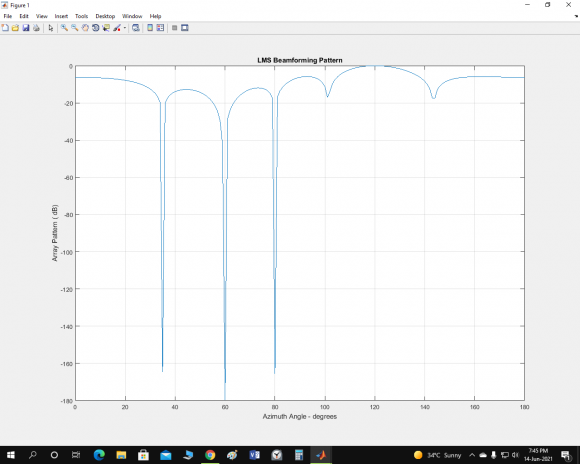## Problem in Beam SteeringStarted by 2 years ago4 replieslatest reply 2 years ago154 views

I am involved in steering the main beam in desired direction and producting nulls in unwanted direction. I have the following MATLAB code but when I run it , it gives me a polar plot. Also it doesn't give me the desired plot. I want to get a plot of type shown in image and to make the pattern such that it meets my requirement.% Generalized Null Steering Broadside Beam former Weights Vector

clear all;close all;clc

a = 4; % Antenna elements

d = 0.5; %Spacing

f = 3e9; % operating frequency

b = input ('The Angle of Incidence of The Desired Source Signal  : ') ;

msgbox ('Enter The Angle of Incidence of The Interference Un-Desired Source Signals Respectively ') ;

pause

for I = 1 : a-1

A(I) = input ('The Angle of Incidence of the Undesired Interference Source Signal : ') ;

end

%Estimation of The Weight Vector of A Null Steering Beamformer

for I = 1 : a

for J = 1 : a

if I ==1

S ( I , J ) = exp ( i*( 2*pi*f*d*( J-1 )*cos ( b ))/( 3*10^8 )) ;

Z ( I ) = 1 ;

else

S ( I , J ) = exp ( i*(2*pi*f*d*( J-1 )*cos ( A(I-1) ))/( 3*10^8 ))  ;

Z ( I )= 0 ;

end

end

end

W = ( pinv ( S ) * Z' ) ;

%Plot of The Output Radiation Pattern

% t = 0 : 0.05 : 2*pi ;

t = 0 : 0.05 : pi ;

M = 0 ;

for I = 1 : a

H = exp ( i*(2*pi*f*d*( I-1 )*cos ( t ))/( 3*10^8 ))  ;

M = M + ( H * W(I) ) ;

end

M = abs ( M ) ;

polar ( t , M , '-r' ) , title ( 'The Generalized Null Steering Beam Former Output Radiation Pattern ') ,

grid on ;

[ - ]make the following changes

% M=abs(M);

M = 20*log10(abs ( M )) ;

%polar ( t , M , '-r' ) ,

plot(t,M,'r')

fred h

[ - ]Thank you very much for your kind response. I made the changes as you directed and ran the program, but it gave me the following error:

To use 'fred', the following product must be both licensed and installed:

Datafeed Toolbox

Error in steerer1 (line 51)

fred h

[ - ]